Question

Given: f(x) = x3+ x2+4x find the following:

Given: f(x) = x3+ x2+4x find the following:

a) Relative extrema b) Table of values c) x-intercepts (if any) d) y-intercept e) Domain f Range g) Interval(s) increasing h) Interval(s) decreasing i) Find All zero s ) Factor completely k) Graph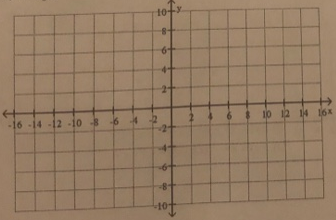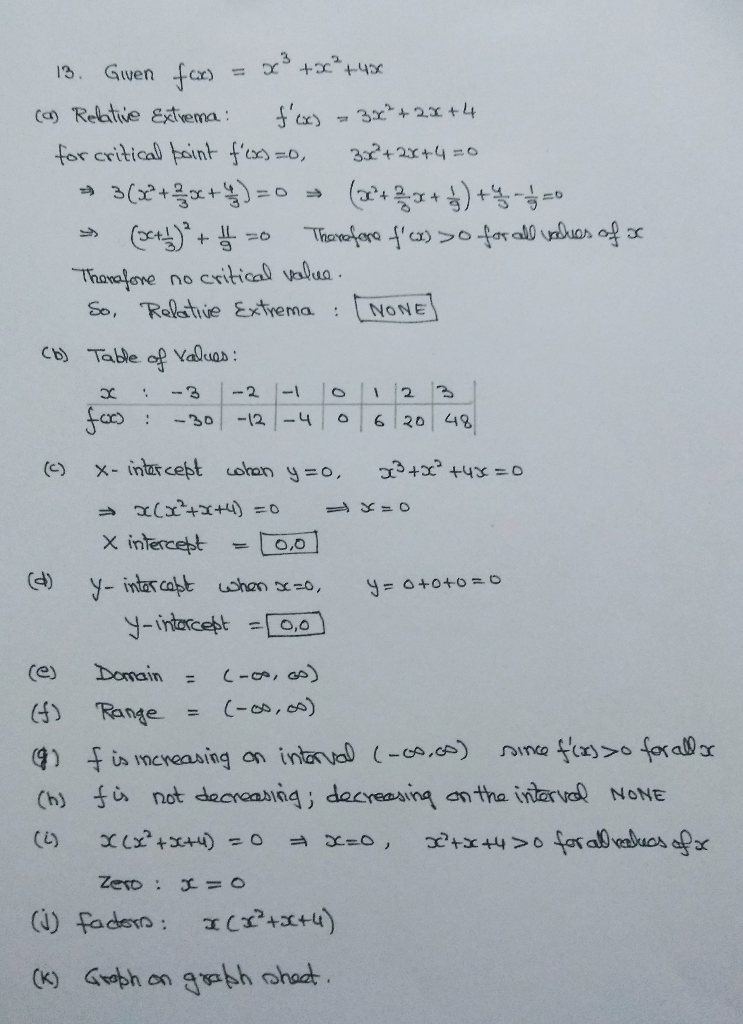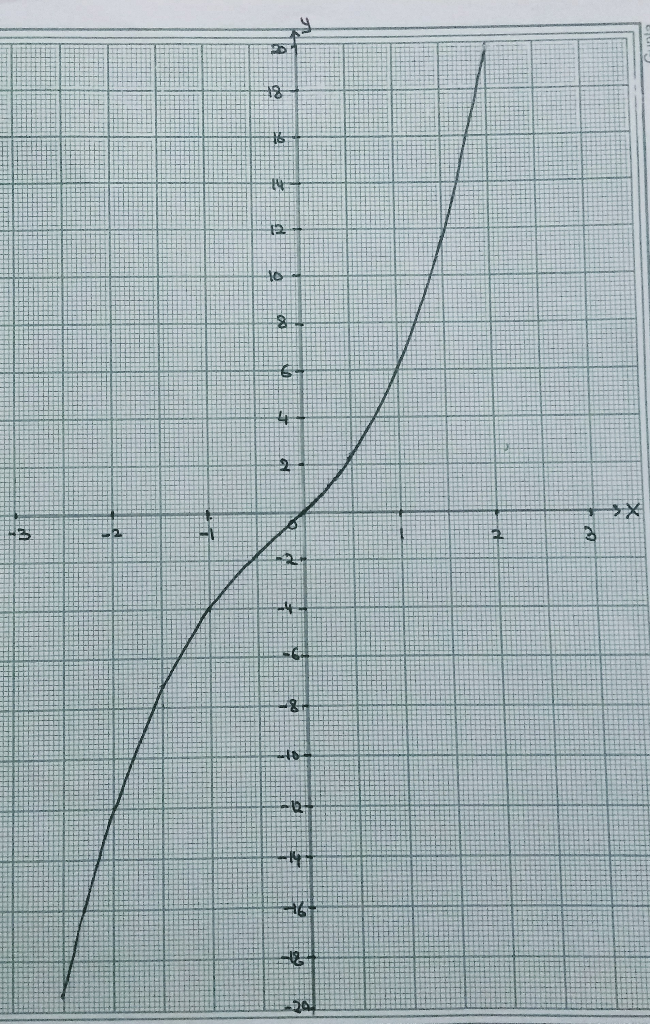Earn Coins

Coins can be redeemed for fabulous gifts.

Similar Homework Help Questions
• For g(x) = 3x2-12x + 6, answer the following questions.

For g(x) = 3x2-12x + 6, answer the following questions. a. Find the values of the x-intercepts b. State the coordinates of the y-intercept. c. State the vertex d. State the axis of symmetry e. State the direction of the parabola. f. Decide whether there is a relative maximum or minimum, then state it. g. Graph the function accurately using the above information. h. State the domain. i. State the range. j. State the interval of x where the graph is increasing k. State the interval of x where the graph...

• 16 For the quadratic function f(x) = -x2 - 8x, answer parts (a) through (c) (a)...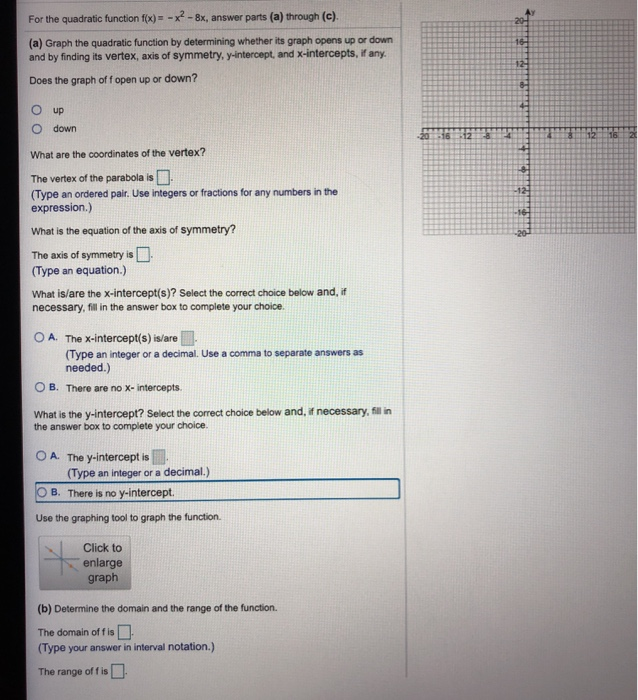16 For the quadratic function f(x) = -x2 - 8x, answer parts (a) through (c) (a) Graph the quadratic function by determining whether its graph opens up or down and by finding its vertex, axis of symmetry, y-intercept, and X-intercepts, if any. Does the graph off open up or down? 12 8- up Odown 2015 12 118 12 16 What are the coordinates of the vertex? The vertex of the parabola is (Type an ordered pair. Use integers or fractions...

• 5. Find where the function f(x) = 2x4 - 4x?- 5 is increasing and decreasing, and...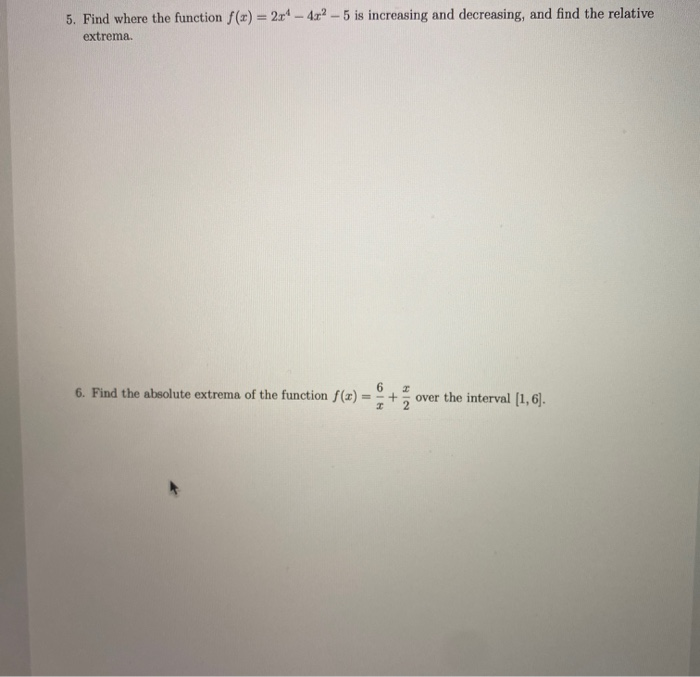5. Find where the function f(x) = 2x4 - 4x?- 5 is increasing and decreasing, and find the relative extrema. 6 6. Find the absolute extrema of the function f(x) + over the interval (1,6]. I

• Name: 1. For the function f(x) = x2 – 1 find and simplify: a. f(-2) b....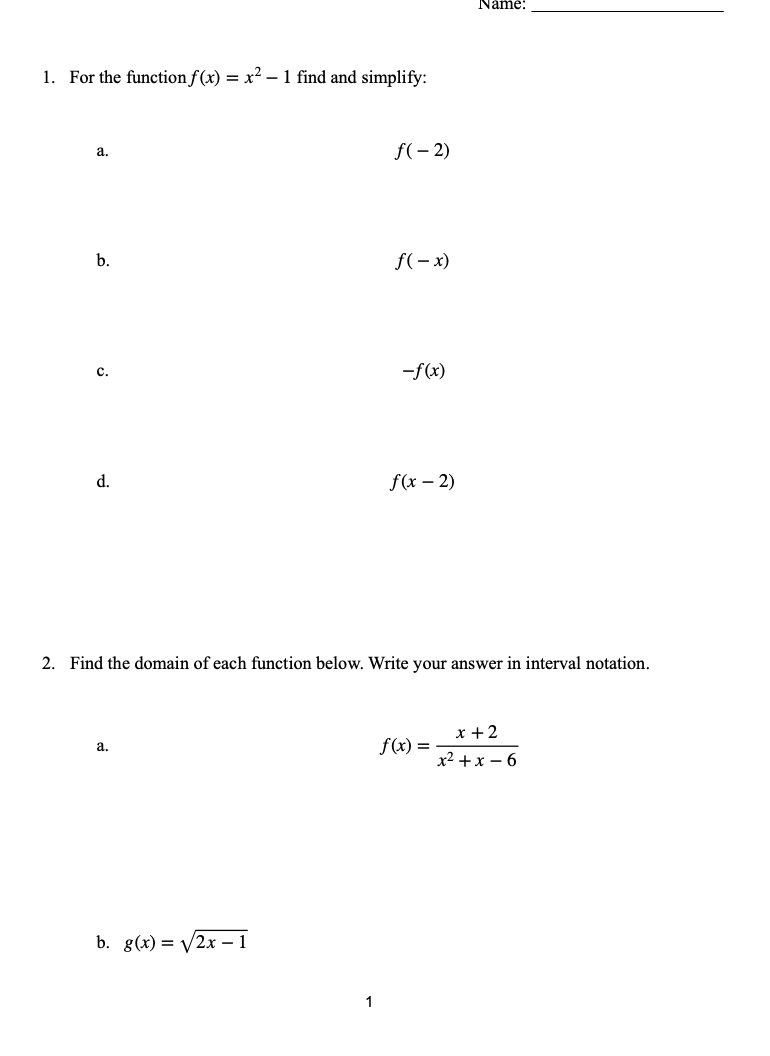Name: 1. For the function f(x) = x2 – 1 find and simplify: a. f(-2) b. f(-x) c. -f(x) d. f(x - 2) 2. Find the domain of each function below. Write your answer in interval notation. a. f(x) = x + 2 x2 + x - 6 b. 8(x) = (2x - 1 1 f(x + h) - f(x) 3. For the function f(x) = 2x2 – 3, find the difference quotient h 4. Use the graph of the...

• A quadratic function f is given. flx) = -x2. 4x 3 (a) Express f in transformation...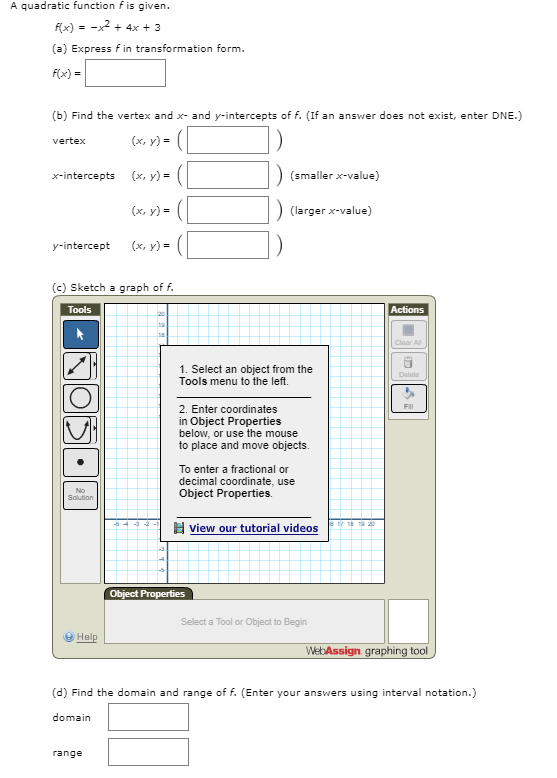A quadratic function f is given. flx) = -x2. 4x 3 (a) Express f in transformation form. f(x) (b) Find the vertex and x and y-intercepts of f. (If an answer does not exist, enter DNE.) (x, y) vertex (x, y) (smaller x-value) x-intercepts (x, y) (larger x-value) (x, y) yintercept (c) Sketch a graph of f. Tools Actions Clear Al 1. Select an object from the Tools menu to the left. Delato 2. Enter coordinates in Object Properties below,...

• The function f is defined as follows. f(x)- 4x-3 if x21 (a) Find the domain of the function. (b) Locate any intercepts....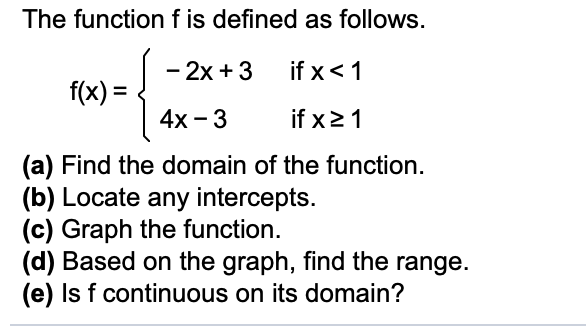The function f is defined as follows. f(x)- 4x-3 if x21 (a) Find the domain of the function. (b) Locate any intercepts. (c) Graph the function. (d) Based on the graph, find the range. (e) Is f continuous on its domain? The function f is defined as follows. f(x)- 4x-3 if x21 (a) Find the domain of the function. (b) Locate any intercepts. (c) Graph the function. (d) Based on the graph, find the range. (e) Is f continuous on...

• 3. Consider the function f(x) = x2 - 6x^2 - 5 a. Find the values of...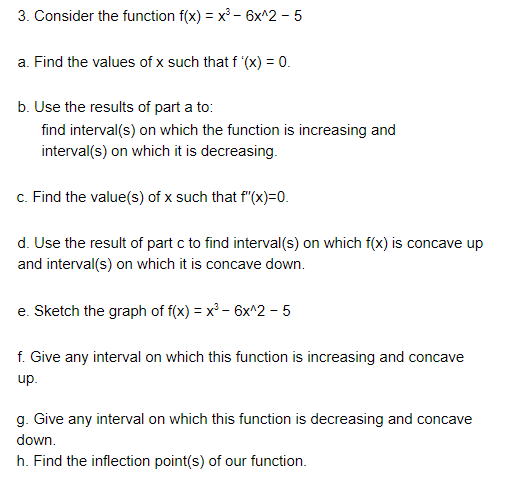3. Consider the function f(x) = x2 - 6x^2 - 5 a. Find the values of x such that f'(x) = 0. b. Use the results of part a to: find interval(s) on which the function is increasing and interval(s) on which it is decreasing. c. Find the value(s) of x such that f"(x)=0. d. Use the result of part c to find interval(s) on which f(x) is concave up and interval(s) on which it is concave down. e. Sketch...

• A Guide to Curve Sketching 1. Determine the domain of f. 2. Find the x- and...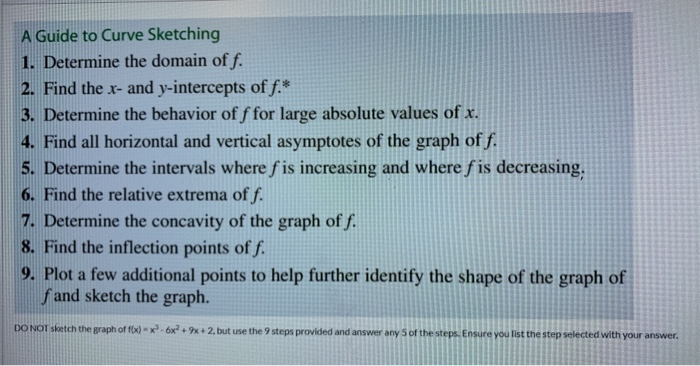A Guide to Curve Sketching 1. Determine the domain of f. 2. Find the x- and y-intercepts off.* 3. Determine the behavior of f for large absolute values of x. 4. Find all horizontal and vertical asymptotes of the graph of f. 5. Determine the intervals where f is increasing and where f is decreasing, 6. Find the relative extrema of f. 7. Determine the concavity of the graph of f. 8. Find the inflection points of f. 9. Plot...

• 3. Use implicit differentiation for the curve x3 + 3y4 = xy and then find the...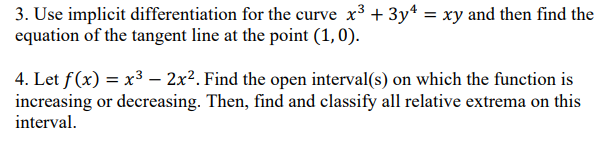3. Use implicit differentiation for the curve x3 + 3y4 = xy and then find the equation of the tangent line at the point (1,0). 4. Let f(x) = x3 – 2x2. Find the open interval(s) on which the function is increasing or decreasing. Then, find and classify all relative extrema on this interval

• graph the quadratic function. Find the x- and y-intercepts of each graph, if any exist. If...

graph the quadratic function. Find the x- and y-intercepts of each graph, if any exist. If it is given in general form, convert it into standard form; if it is given in standard form, convert it into general form. Find the domain and range of the function and list the intervals on which the function is increasing or decreasing. Identify the vertex and the axis of symmetry and determine whether the vertex yields a relative and absolute maximum or minimum....